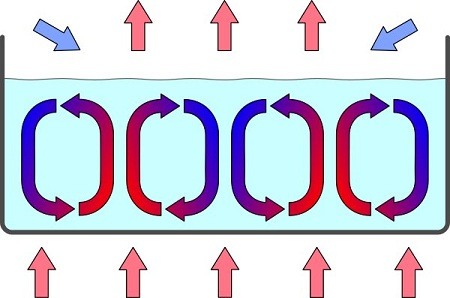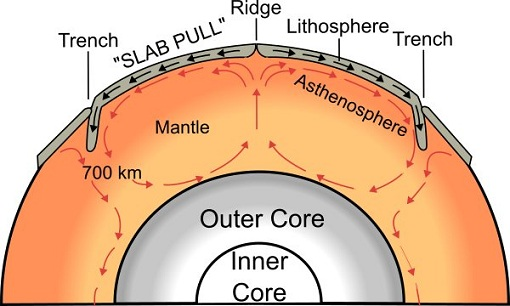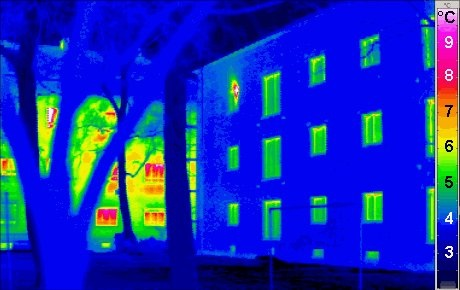# Difference Between Convection and Radiation

## Main Difference – Convection vs. Radiation

Convection and radiation are both mechanisms of heat transfer. They allow thermal energy to be transported from one place to another. The main difference between convection and radiation is that convection is a mechanism of heat transfer which involves a mass flow of material. Radiation, on the other hand, is a transfer of heat using electromagnetic energy. Consequently, radiation can transfer heat through a vacuum.

## What is Convection

Convection is the mechanism of heat transfer in materials through mass flow of the material. In order to conduct heat, parts of the material itself moves — i.e. there is a transfer of mass within the material. Typically, convection occurs in fluids. However, effects of convection can be seen sometimes in solids, as in the case of plate tectonics. There are two main types of convection: natural and forced.

Convection is a complex process and there is no simple equation that fully describes it. However, we can make use of an approximation for cases where a fluid is heated using a solid surface. For these cases, the heat transfer rate$\frac{\mathrm{d}Q}{\mathrm{d}t}$ is given by,$\frac{\mathrm{d}Q}{\mathrm{d}t}=hA\left( T_s-T_a\right)$

where$A$ is the surface area that heat is transferred through,$T_s$ is the temperature of the solid,$T_a$ is the temperature of air.$h$ is known as the convective heat transfer coefficient. This coefficient depends on a number of properties including the density, viscosity, and the flow rate of the fluid.

## Natural Convection

In natural convection, flow of materials is caused by differences in density. For instance, let us consider a kettle of water being warmed up on a stove. As the water heats up at the bottom of the kettle, it expands. This means that the water molecules are now placed further apart, which causes the density of water at the bottom to decrease. Now, the water at the bottom of the kettle is less dense compared to the water at the top of the kettle. Due to the density difference, the warmer water from the bottom rises to the top while the colder water from the top sinks to the bottom. The process repeats until the top and bottom are both at the same temperature.

The rising hot fluid cannot rise along the same line where the cold fluid is sinking. Therefore, the fluid needs to move horizontally before rising/sinking for the next cycle. This sets up convection cells in the fluid, as shown in the diagram below.Convection Cells

Natural convection is responsible for air currents and it is also one of the main factors involved in ocean currents.

Convection is also an important factor in plate tectonics. The inner parts of the Earth’s mantle is hotter than the outer part, and this causes convection cells to be set up in the mantle. The mantle is solid, and the movement of material within the mantle is quite slow, about 20 mm per year.Convection in the Earth’s Mantle

## Forced Convection

Forced convection occurs when the movement of material is moved using an external mechanism such as using a fan or a pump. Fan heaters are a good example for forced convection. In the human body, the heart also acts as a pump responsible for forced convection of heat around the body.

Radiation describes the transfer of heat via electromagnetic radiation. Due to kinetic energy, molecules that make up objects are always in motion. This causes charges in those molecules to move, which results in the creation of electromagnetic waves.

The rate at which an object emits heat through radiation is given by the Stefan-Boltzmann law:$\frac{\mathrm{d}Q}{\mathrm{d}t}=\epsilon \sigma AT^4$

where$A$ is the surface area of the object and$T$ is its absolute temperature.$\sigma$ is the Stefan-Boltzmann constant,$\sigma=\mathrm{5.670\times 10^{-8}\:W\:m^{-2}\:K^{-4}}$.

The quantity$\epsilon$ is called emissivity. It takes a value between 0 and 1.$\epsilon$ is higher for darker objects with darker surfaces, which emit and absorb radiation well. Shiny surfaces absorb and emit much less radiation and they have emissivities closer to 0. An ideal surface that is both a perfect absorber and an emitter of radiation has an emissivity of 1 and is called a blackbody.

As the object is emitting radiation to surroundings, it is also absorbing radiation from surroundings. If the surroundings are at a temperature of$T_s$, the net rate at which a body radiates heat is given by${\left( \frac{\mathrm{d}Q}{\mathrm{d}t}\right)}_{net}=\epsilon \sigma A{\left( T^4-T_s^4\right)}$

If$T>T_s$ there is a net heat radiation is from the body to the surroundings.

Objects emit some wavelengths of radiation more than others. Typically, the hotter the body, the lower the wavelength that is emitted the most. For instance, hotter stars should have a bluer colour (smaller wavelength) compared to colder, redder ones (larger wavelength). For an ideal blackbody at an absolute temperature$T$Wien’s law gives the wavelength$\lambda_{max}$ which is emitted the most:$\lambda_{max}T=2.898\times10^{-3}\mathrm{\:m\:K}$

At room temperature, the primary wavelength radiated by bodies is in the infra-red range. The graph below shows the energy density of a given wavelength radiated by a blackbody at several different temperatures.Thermograms make use of thermal radiation emitted by the body to screen diseases and infra-red cameras are used to “see” in the dark. Radiation from distant stars is also used to measure the distance between the Earth and the stars.Difference Between Convection and Radiation – Thermogram of an Energy-efficient house in the foreground, radiating much less thermal energy compared to a traditional house radiating much more energy (background)

## What is the difference between Convection and Radiation

### Origin

Convection takes place as a result of thermal expansion of matter.

Radiation is a result of the motion of charges in materials due to kinetic energy of the molecules.

### Mechanism

Convection involves a transfer of mass of a material, typically a fluid.

Radiation involves an electromagnetic wave. Matter itself does not move.

### Medium

Convection requires a medium.

Radiation does not require a medium, and can transfer heat through a vacuum.

### Temperature Dependence

Convection results in a heat flow rate that is approximately directly proportional to the temperature difference.

Radiation results in a heat flow rate that is dependent on the difference between  fourth powers of temperatures of the object and the surroundings.

###### “Thermogram of a Passivhaus building, with traditional building in background.” by Passivhaus Institut (Copied to Commons from http://en.wikipedia.org. Original source Passivhaus Institut, Germany – http://www.passiv.de)  [CC BY-SA 3.0], via Wikimedia Commons